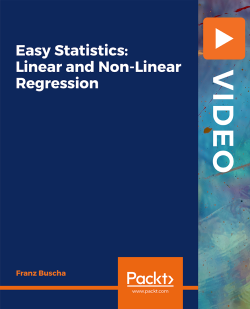# Easy Statistics: Linear and Non-Linear Regression [Video]

By Franz Buscha
• \$30.99 Video
• \$12.99 Video + Subscription
What do you get with a Packt Subscription?

• Constantly updated with 100+ new titles each month
• Breadth and depth in over 1,000+ technologies

Working with statistics and quantitative reports requires a good understanding of statistics fundamentals and techniques. However, learning and applying new statistical techniques can often be a daunting experience. This is where this course comes into play.

To make your experience with statistics a pleasant one, this course gives you comprehensive knowledge of basic principles of the statistical methodology, focusing on linear regression and non-linear regression.

The course starts with an introduction to easy statistics and gives you an overview of the course objectives. Next, you will explore the types of regression analysis that exist and find out how ordinary least squares (OLS) works. To gain a deeper understanding of linear regression and OLS, you will learn to interpret and analyze complicated regression output from OLS. You will also focus on Gauss–Markov assumptions and zero conditional mean. Moving ahead, you will cover non-linear regression, exploring how it works, what the different non-linear regression models are, and the major uses. Towards the end, you will learn to work around with regression modeling with the help of practical examples.

By the end of this video, you will be well-versed with linear and non-linear regression and the basic principles of the statistical methodology.

All the resource files are added on GitHub at https://github.com/PacktPublishing/-Easy-Statistics-Linear-and-Non-Linear-Regression

Publication date:
November 2020
Publisher
Packt
Duration
5 hours 16 minutes
ISBN
9781800566590